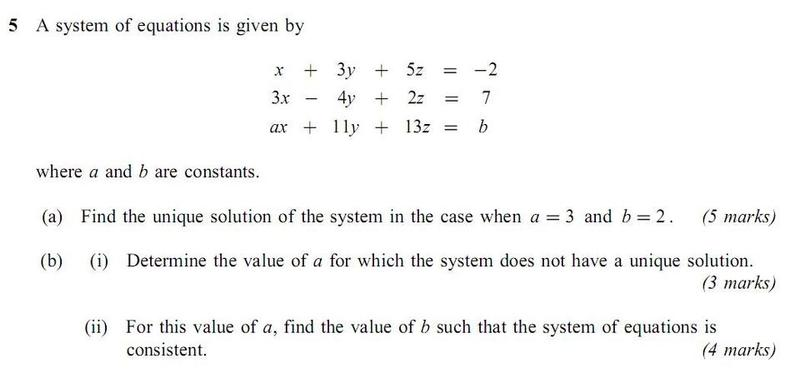# Constants in a system of equations that makes the system consistent

## Homework Statement## The Attempt at a Solution

For a) I just solved simultaneous equations, a link to a resource that solves a system more efficiently would be nice but augmented matrices are taught in the syllabus, or atleast not in the official text. Although I've seen solutions in the mark schemes of augmented matrices.

Next I get the determinent in terms of a, a=1 when determinent is 0.

I'm not sure how to do this last part.

Pengwuino
Gold Member
If you conduct row operations, you would have gotten the same result for part b) but in the form of a row being something like (a-*)x + 0y + 0z = b+** with * and ** being whatever numbers you actually get, I didn't calculate it. Well what you found was that * was equal to 1 to make it 0x + 0y + 0z = something which was inconsistent and had no solution. However, that "something" which is a function of b can be made to be 0 depending on what you pick for b. That is, you'd get 0x + 0y + 0z = 0 which is now a consistent system

EnumaElish
Homework Helper
When a = 1, does the system have any solution?

Is that what they meant by "does not have a unique solution"?

When a = 1, does the system have any solution?

Is that what they meant by "does not have a unique solution"?

No solutions or infinite solutions i.e. a line of solutions in the intersection of two planes.

Pengwuino
Gold Member
I assume the spirit of the problem is to first find a "no solution" then an infinite solution in part ii) since in part i), you'll have b that isn't known yet.

I'm trying to work out how to do what Pengwuino said for me to do.

Mark44
Mentor
To summarize part of what Pengwuino said, after row reducing the augmented matrix [A|b], where A represents your matrix of coefficients, and b represents the vector of constants on the right sides of the equations, you're looking to get one or more rows of zeroes in the bottom of A.

There are two possibilities:

1. 0 0 0 | k , where k != 0
This represents 0x + 0y + 0z = k, for which there is no solution.
2. 0 0 0 | 0
This represents 0x + 0y + 0z = 0, for which there are an infinite number of solutions.